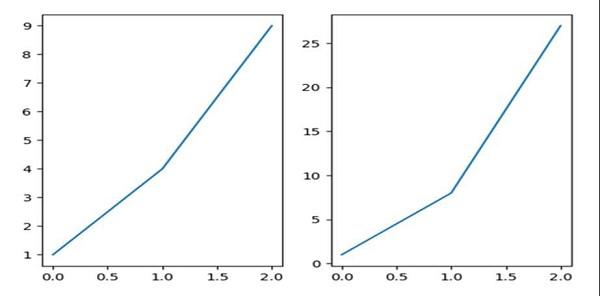# How to zoom subplots together in Matplotlib/Pyplot?

We can use the attribute sharex = "ax1", and then, use the subplot method to zoom the subplots together.

## Steps

• Add a subplot to the current figure with (nrow = 1, ncols = 2, index = 1).

• Add line on the current subplot with (nrow = 1, ncols = 2, index = 1).

• Add a subplot to the current figure with (nrow = 1, ncols = 2, index = 2).

• Add line on the current subplot with (nrow = 1, ncols = 2, index = 2), where sharex can help to share the x or y ~matplotlib.axis with sharex and/or sharey. The axis will have the same limits, ticks, and scale as the axis of the shared axes.

• Using plt.show(), show the figures.

## Example

from matplotlib import pyplot as plt
ax1 = plt.subplot(1, 2, 1)
ax1.plot([1, 4, 9])
ax2 = plt.subplot(1, 2, 2, sharex=ax1)
ax2.plot([1, 8, 27])
plt.show()

## Output